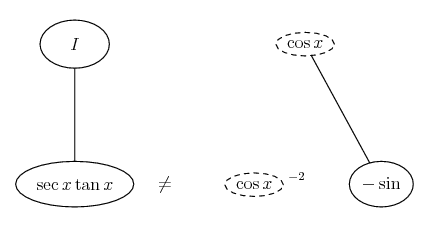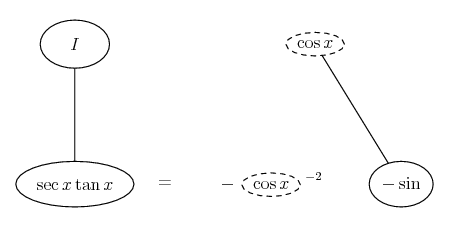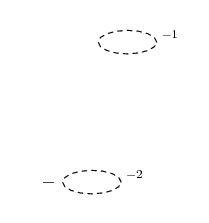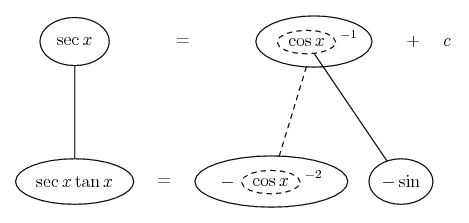# Thread: Integral Help

1. ## Integral Help

I am having the hardest time trying to integrate the following problem.

$\displaystyle \int\left[sec(x)*tan(x)\right]dx$

I have tried writing the problem using sines and cosines but that did not help me. In my book it says the integral of this problem is sec(x), but I cannot get it.My book also gives me tips on how to integrate this problem when it sec(x) and tan(x) are raised to powers greater than one. If anyone can help me out I would greatly appreciate it.

2. Consider the derivative of $\displaystyle \sec{x}$

$\displaystyle (\sec{x})'$

$\displaystyle = ( \frac{1}{\cos{x}})'$

$\displaystyle = \frac{ - (- \sin{x})}{ \cos^2{x} }$

$\displaystyle = \frac{ \tan{x}}{\cos{x}}$

$\displaystyle = \sec{x} \tan{x}$

3.Originally Posted by fishgutsI am having the hardest time trying to integrate the following problem.

$\displaystyle \int\left[sec(x)*tan(x)\right]dx$

I have tried writing the problem using sines and cosines but that did not help me. In my book it says the integral of this problem is sec(x), but I cannot get it.My book also gives me tips on how to integrate this problem when it sec(x) and tan(x) are raised to powers greater than one. If anyone can help me out I would greatly appreciate it.
$\displaystyle \int\left[sec(x)*tan(x)\right]dx$
=$\displaystyle \int\frac{sinx}{cos^2x}dx$
for integrating, substitute $\displaystyle cosx=t$

4.Originally Posted by simplependulumConsider the derivative of $\displaystyle \sec{x}$

$\displaystyle (\sec{x})'$

$\displaystyle = ( \frac{1}{\cos{x}})'$

$\displaystyle = \frac{ - (- \sin{x})}{ \cos^2{x} }$

$\displaystyle = \frac{ \tan{x}}{\cos{x}}$

$\displaystyle = \sec{x} \tan{x}$

hmm. I can kind of see how to do the problem now, by working backwards a bit. When I am first starting the problem though would I make a substitution of some sort?

5. Differentiation and integration are inverse operations (up to a constant). So if you know the function whose derivative is secxtanx, then you know the indefinite integral of secxtanx.

6.Originally Posted by fishgutsI have tried writing the problem using sines and cosines but that did not help me.
To be fair, it helped alot! Once you have the integrand as...

$\displaystyle \sin x \cos^{-2} x$

... then you can 'spot the derivative' - sin being roughly the derivative of cos, which is then a very likely inner function of a chain rule process. Not precisely the derivative, of course - actually minus sin...... so fix things...... so that the lower equals sign is valid. Then integrate with respect to the dashed balloon expression (or u- sub)...... which we indicate in balloon world with a straight dashed line.Don't integrate - balloontegrate!

Balloon Calculus Forum

#### Search Tags

integral